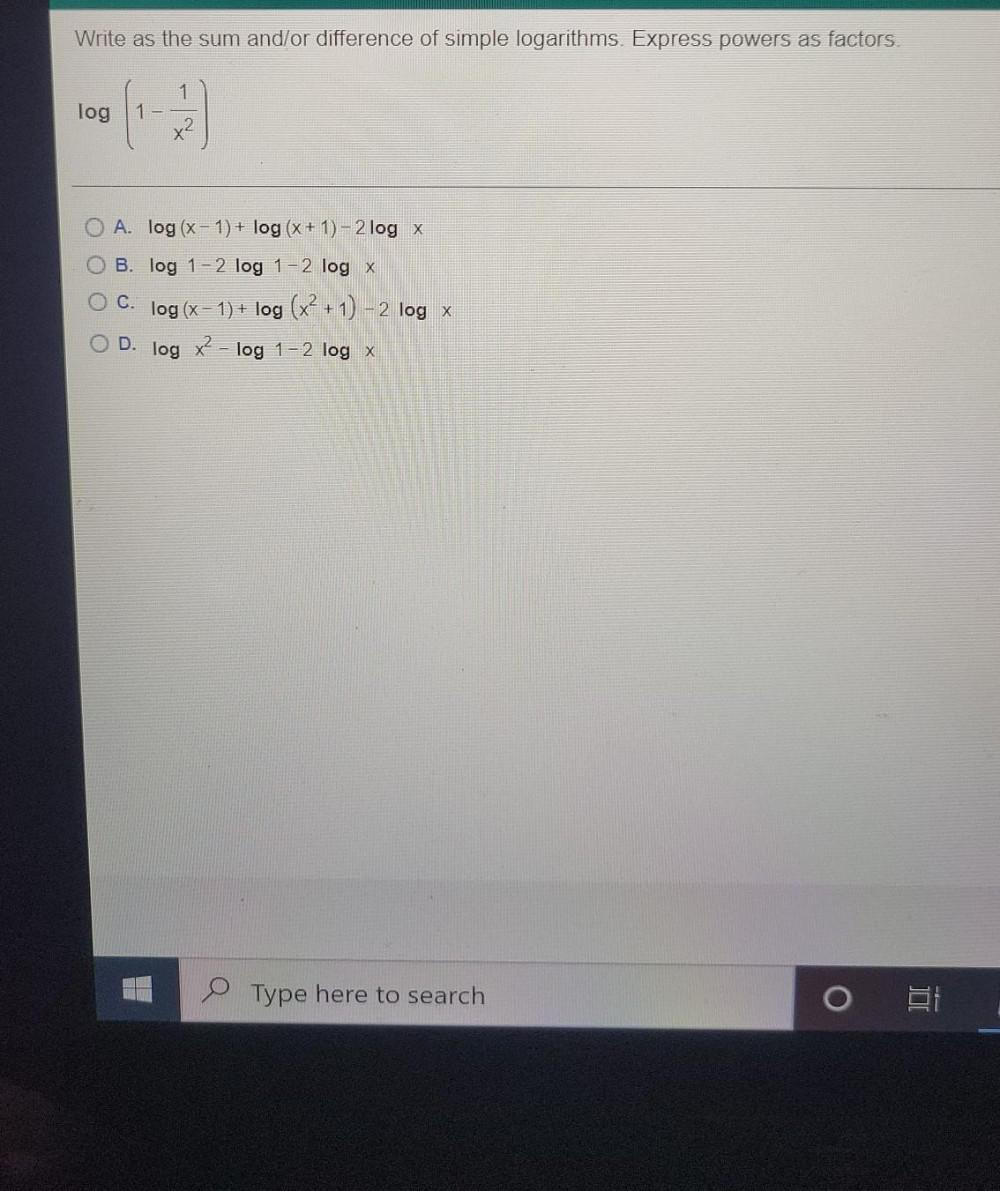Question:

# Write as the sum and/or difference of simple logarithms. Express powers as factors log 1 x2 A. log (x - 1)+ log (x + 1) - 2 logWrite as the sum and/or difference of simple logarithms. Express powers as factors log 1 x2 A. log (x - 1)+ log (x + 1) - 2 log x B. log 1-2 log 1-2 log X O C. log (x - 1) + log (x + 1) - 2 log x D. log x2 - - log 1 - 2 log X O Type here to search o E